# NCERT Solutions for Class 6 Maths Chapter 8 Decimals in Hindi

## NCERT Solutions for Class 6 Maths Chapter 8 Decimals in Hindi PDF Download

Download the Class 6 Maths NCERT Solutions in Hindi medium and English medium as well offered by the leading e-learning platform Vedantu. If you are a student of Class 6, you have reached the right platform. The NCERT Solutions for Class 6 Maths in Hindi provided by us are designed in a simple, straightforward language, which are easy to memorise.

You will also be able to download the PDF file for NCERT Solutions for Class 6 Maths in English and Hindi from our website at absolutely free of cost. Register Online for NCERT Class 6 Science tuition on Vedantu.com to score more marks in CBSE board examination.

NCERT, which stands for The National Council of Educational Research and Training, is responsible for designing and publishing textbooks for all the classes and subjects. NCERT textbooks covered all the topics and are applicable to the Central Board of Secondary Education (CBSE) and various state boards.

We, at Vedantu, offer free NCERT Solutions in English medium and Hindi medium for all the classes as well. Created by subject matter experts, these NCERT Solutions in Hindi are very helpful to the students of all classes.

Do you need help with your Homework? Are you preparing for Exams?
Study without Internet (Offline)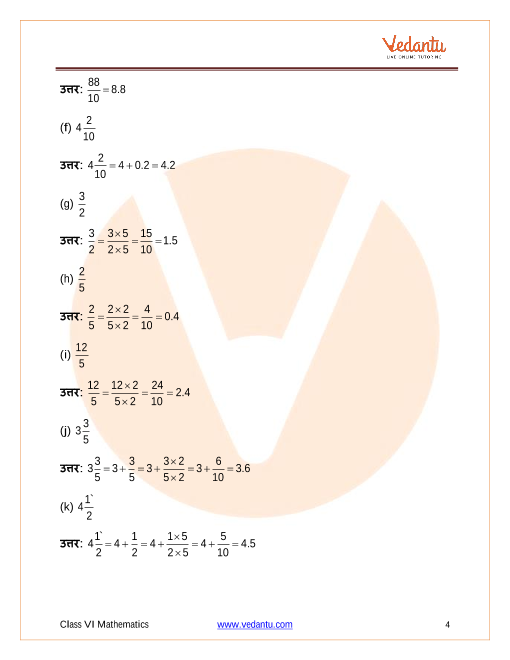Book your Free Demo session
Get a flavour of LIVE classes here at Vedantu## NCERT Solutions for Class 6 Maths Chapter 8 Decimals in Hindi

### प्रश्नावली 8.1

1: निम्न के लिए दी गई सारणी में संख्याएँ लिखिए: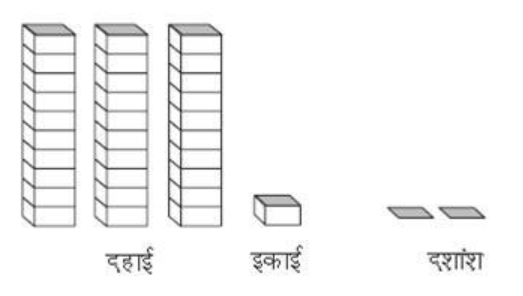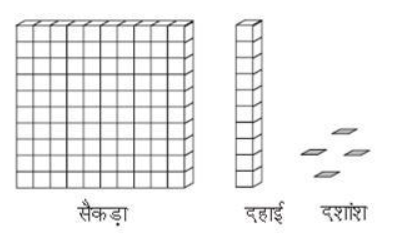उत्तर:

 सैकड़ा 100 दहाई 10 इकाई1 दशांश($\frac{1}{10}$) $0$ $1$ $9$ $4$ $0$ $3$ $1$ $31.2$ $1$ $1$ $0$ $110.4$

2: निम्न दशमलव संख्याओं को स्थानीय मान सारणी में लिखिए:

1. 19.4

2. 0.3

3. 10.6

4. 205.9

उत्तर:

 सैकड़ा 100 दहाई 10 इकाई1 दशांश($\frac{1}{10}$) a. $0$ $1$ $9$ $4$ b. $0$ $0$ $0$ $3$ c. $0$ $1$ $0$ $6$ d. $2$ $0$ $5$ $9$

3: निम्न में से प्रत्येक को दशमलव के रूप में लिखिए:

$\left( \mathbf{a} \right)7$ दशांश

उत्तर- $7$ दशांश $=\frac{7}{10}=0.7$

(b) $2$ दहाई, $9$दशांश

उत्तर-$2$ दहाई, $9$दशांश $=2\times 10+\frac{9}{10}=20+0.9=20.9$

(c)चौदह दशमलव छ:

उत्तर: चौदह दशमलव छ:$=14.6$

(d)एक सौ और दो ईकाई

उत्तर: एक सौ और दो ईकाई $=100+2\times 1=102$

(e)छ: सौ दशमलव आठ

उत्तर: छ: सौ दशमलव आठ$=600.8$

4: निम्न को दशमलव रूप में व्यक्त कीजिए:

1. $\mathbf{\frac{5}{10}}$

उत्तर:  $\frac{5}{10}=0.5$

1. $\mathbf{3+\frac{7}{10}}$

उत्तर: $3+\frac{7}{10}=3+0.7=3.7$

1. $\mathbf{200+60+5+\frac{1}{10}}$

उत्तर: $200+60+5+\frac{1}{10}=200+60+5+0.1=265.1$

1. $\mathbf{70+\frac{8}{10}}$

उत्तर: $70+\frac{8}{10}=70+0.8=70.8$

1. $\mathbf{\frac{88}{10}}$

उत्तर: $\frac{88}{10}=8.8$

1. $\mathbf{4\frac{2}{10}}$

उत्तर: $4\frac{2}{10}=4+0.2=4.2$

1. $\mathbf{\frac{3}{2}}$

उत्तर: $\frac{3}{2}=\frac{3\times 5}{2\times 5}=\frac{15}{10}=1.5$

1. $\mathbf{\frac{2}{5}}$

उत्तर: $\frac{2}{5}=\frac{2\times 2}{5\times 2}=\frac{4}{10}=0.4$

1. $\mathbf{\frac{12}{5}}$

उत्तर: $\frac{12}{5}=\frac{12\times 2}{5\times 2}=\frac{24}{10}=2.4$

1. $\mathbf{3\frac{3}{5}}$

उत्तर: $3\frac{3}{5}=3+\frac{3}{5}=3+\frac{3\times 2}{5\times 2}=3+\frac{6}{10}=3.6$

1. $\mathbf{4\frac{1\grave{\ }}{2}}$

उत्तर: $4\frac{1\grave{\ }}{2}=4+\frac{1}{2}=4+\frac{1\times 5}{2\times 5}=4+\frac{5}{10}=4.5$

5: निम्न दशमलव संख्याओं को भिन्न के रूप में लिखकर न्यूनतम (सरलतम) रूप में बदलिए:

1. 0.6

उत्तर: $0.6=\frac{6}{10}=\frac{3}{5}$

1. 2.5

उत्तर: $\text{ }2.5=\frac{25}{10}=\frac{5}{2}$

1. 1.0

उत्तर: 1

1. 3.8

उत्तर: $3.8=\frac{38}{10}=\frac{19}{5}$

1. 13.7

उत्तर: $13.7=\frac{137}{10}$

1.  21.2

उत्तर: $21.2=21+\frac{2}{10}=21+\frac{1}{5}=21\frac{1}{5}=\frac{106}{5}$

1. 6.4

उत्तर: $6.4=6+\frac{4}{10}=6+\frac{2}{5}=6\frac{2}{5}$

6: सेमी का प्रयोग कर निम्न को दशमलव रूप में बदलिए:

1. $\mathbf{2}$ मिमी

उत्तर: चूकी $10$ मिमी$=1$सेमी

इस प्रकार, $1$ मिमी$=$$\frac{1}{10}$ सेमी

$2$ मिमी$=$$\frac{1}{10}\times 2=0.2$ सेमी

1. 30 मिमी

उत्तर: चूकी $\ 10$मिमी$=1$सेमी

इस प्रकार, $1$ मिमी$=$$\frac{1}{10}$ सेमी

$30$ मिमी=$\frac{1}{10}\times 30=3.0$ सेमी

1. 116 मिमी

उत्तर: चूकी $10$मिमी$=1$सेमी

इस प्रकार, $1$ मिमी$=$$\frac{1}{10}$ सेमी

$116$मिमी$=\frac{1}{10}\times 116=11.6$ सेमी

1. $\mathbf{4}$ सेमी $\mathbf{2}$ मिमी

उत्तर: चूकी $10$मिमी$=1$सेमी

इस प्रकार, $1$ मिमी$=$$\frac{1}{10}$ सेमी

$\mathbf{4}$ सेमी +$\mathbf{2}$ मिमी $=4+\frac{1}{10}\times 2= 4.2$ सेमी

1. 11 सेमी 52मिमी

उत्तर: चूकी $10$मिमी$=1$सेमी

इस प्रकार,$1$ मिमी$=$$\frac{1}{10}$ सेमी

$11$ सेमी $52$मिमी =$=11+\frac{1}{10}\times 52=16.2$ सेमी

1. 83 मिमी

उत्तर: चूकी $10$ मिमी $=1$सेमी

इस प्रकार, $1$ मिमी$=$$\frac{1}{10}$ सेमी

$83$ मिमी=$=\frac{1}{10}\times 83=8.3$ सेमी

7: संख्या रेखा पर किन दो पूर्ण संख्याओं के बीच निम्न संख्याएँ स्थित हैं? इनमें से कौन सी पूर्ण संख्याएँ दी हुई दशमलव संख्या के अधिक निकट है?
$\left( a \right)\text{ }0.8\text{ }\left( b \right)\text{ }5.1\text{ }\left( c \right)\text{ }2.6\text{ }\left( d \right)\text{ }6.4\text{ }\left( e \right)\text{ }9.0\text{ }\left( f \right)\text{ }4.9$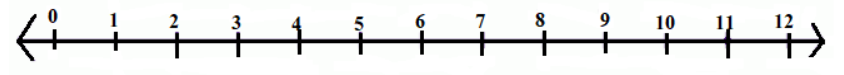उत्तर:

1. 0 और 1के बीच और 1 के निकट

2. 5 और 6 के बीच और 5 के निकट

3. 2 और 3 के बीच और 3 के निकट

4. 6और 7 के बीच और 6 के निकट

5. यह एक पूर्ण संख्या है

6. 4 और 5 के बीच और 5 के निकट

8: निम्न को संख्या रेखा पर दर्शाओ:

$(a)\text{ }0.2\text{ }\left( b \right)\text{ }1.9\text{ }\left( c \right)\text{ }1.1\text{ }\left( d \right)\text{ }2.5$

उत्तर: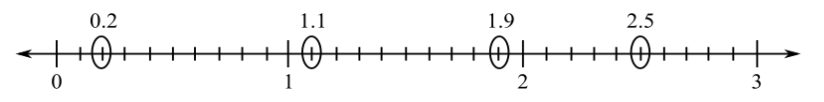9: दी हुई संख्या रेखा पर स्थित $\mathbf{A},\text{ }\mathbf{B},\text{ }\mathbf{C},\text{ }\mathbf{D}$ बिन्दुओं के लिए दशमलव संख्या लिखिए: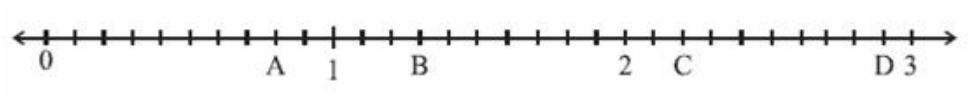उत्तर: $\left( A \right)\text{ }0.8\text{ }\left( B \right)\text{ }1.3\text{ }\left( C \right)\text{ }2.2\text{ }\left( D \right)\text{ }2.9$

10:

1. रमेश के कॉपी की लंबाई $\mathbf{9}$ सेमी $\mathbf{5}$ मिमी है। सेमी में इसकी लंबाई क्या होगी?

उत्तर: हम जानते हैं कि $1$मिमी = $0.1$सेमी

इसलिए 5 मिमी $=0.5$सेमी

इसलिए $9$ सेमी $+5$ मिमी $=9.5$सेमी

1. चने के एक छोटे पौधे की लंबाई $\mathbf{65}$ मिमी है। इसकी लंबाई सेमी में व्यक्त कीजिए।

उत्तर: हम जानते हैं कि $1$मिमी = $0.1$सेमी

$65$ मिमी $=6.5$सेमी

### प्रश्नावली 8.2

1: इन बक्सों की सहायता से सारणी को पूरा कर दशमलव रूप में लिखिए: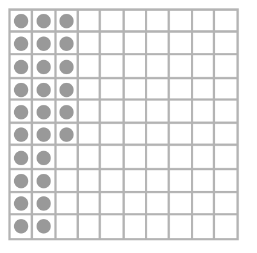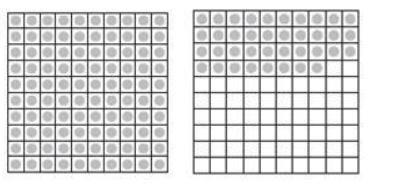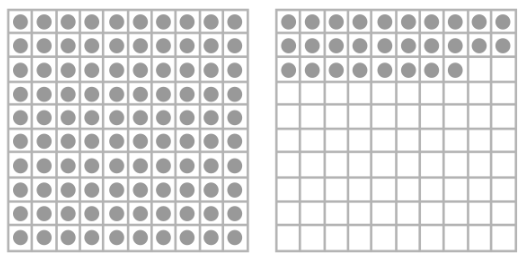उत्तर:

 इकाई दहाई शतांश अंक (a) $0$ $2$ $6$ $0.26$ (b) $1$ $3$ $8$ $1.38$ (c) $1$ $2$ $8$ $1.28$

2 : स्थानीय मान सारणी को देखकर दशमलव रूप में देखिए:

 सैकड़ा100 दहाई10 इकाई1 दशांश$\mathbf{\frac{1}{10}}$ शतांश$\mathbf{\frac{1}{100}}$ हजारवाँ$\mathbf{\frac{1}{1000}}$ (a) 0 0 3 2 5 0 (b) 1 0 2 6 3 0 (c) 0 3 0 0 2 5 (d) 2 1 1 9 0 2 (e) 0 1 2 2 4 1

उत्तर: $\left( a \right)\text{ }3.25\text{ }\left( b \right)\text{ }102.63\text{ }\left( c \right)\text{ }30.025\left( d \right)\text{ }211.902\text{ }\left( e \right)\text{ }12.241$

3: निम्न दश्मलवों को स्थानीय मान सारणी बनाकर लिखिए:
$\left( a \right)0.29\text{ }\left( b \right)\text{ }2.08\text{ }\left( c \right)\text{ }19.60\text{ }\left( d \right)\text{ }148.32\text{ }\left( e \right)\text{ }200.812$

उत्तर:

 संख्या सैकड़ा100 दहाई10 इकाई1 दशांश$\mathbf{\frac{1}{10}}$ शतांश$\mathbf{\frac{1}{100}}$ हजारवाँ$\mathbf{\frac{1}{1000}}$ (a) $\mathbf{0}.\mathbf{29}$ $0$ $0$ $0$ $2$ $9$ $0$ (b) $\mathbf{2}.\mathbf{08}$ $0$ $0$ $2$ $0$ $8$ $0$ (c) $19.60$ $0$ $1$ $9$ $6$ $0$ $0$ (d) $148.32$ $1$ $4$ $8$ $3$ $2$ $0$ (e) $200.812$ $2$ $0$ $0$ $8$ $1$ $2$

4: निम्न में से प्रत्येक को दशमलव रूप में लिखिए:

1. $\mathbf{20+9+\frac{4}{10}+\frac{1}{100}}$

2. $\mathbf{137+\frac{5}{100}}$

3. $\mathbf{\frac{7}{10}+\frac{6}{100}+\frac{4}{1000}}$

4. $\mathbf{23+\frac{2}{10}+\frac{6}{1000}}$

5. $\mathbf{700+20+5+\frac{9}{100}}$

उत्तर:

1. $20+9+0.4+0.01=29.41$

2. $137+0.05=137.05$

3. $0.7+0.06+0.004=0.764$

4. $23+0.2+0.006=23.206$

5. $700+20+5+0.09=725.05$

5: निम्न दशमलवों को शब्दों में लिखिए:

1. 0.03

उत्तर: शून्य दशमलव शून्य तीन

1. 1.20

उत्तर: एक दशमलव दो

1. 108.56

उत्तर: एक सौ आठ दशमलव पाँच छ:

1. 10.07

उत्तर: दस दशमलव शून्य सात

1. 0.032

उत्तर: शून्य दशमलव शून्य तीन दो

1. 5.008

उत्तर: पाँच दशमलव शून्य शून्य आठ

6: संख्या रेखा के किन दो बिंदुओं के बीच निम्न संख्याएँ स्थित हैं?

x$\left( a \right)\text{ }0.06\text{ }\left( b \right)\text{ }0.45\text{ }\left( c \right)\text{ }0.19\text{ }\left( d \right)\text{ }0.66\text{ }\left( e \right)\text{ }0.92\text{ }\left( f \right)\text{ }0.57$

उत्तर: सभी संख्याये 0 और 1 के बीच स्थित है|

1. 0 और 0.1

2. 0.4और 0.5

3. 0.1और 0.2

4. 0.6और 0.7

5. 0.9और 1

6. 0.5और 0.6

7: न्यूनतम रूप में भिन्न बनाकर लिखिए:

$\left( \mathbf{a} \right)\text{ }\mathbf{0}.\mathbf{60}$

उत्तर: $0.60=\frac{60}{100}=\frac{3}{5}$

$\left( \mathbf{b} \right)\text{ }\mathbf{0}.\mathbf{05}$

उत्तर: $0.05=\frac{5}{100}=\frac{1}{20}$

$\left( \mathbf{c} \right)\text{ }\mathbf{0}.\mathbf{75}$

उत्तर: $0.75=\frac{75}{100}=\frac{3}{4}$

$\left( \mathbf{d} \right)\text{ }\mathbf{0}.\mathbf{18}$

उत्तर: $0.18=\frac{18}{100}=\frac{9}{50}$

$\left( \mathbf{e} \right)\text{ }\mathbf{0}.\mathbf{25}$

उत्तर: $0.25=\frac{25}{100}=\frac{1}{4}$

$\left( \mathbf{f} \right)\text{ }\mathbf{0}.\mathbf{125}$

उत्तर: $0.125=\frac{125}{1000}=\frac{1}{8}$

$\left( \mathbf{g} \right)\text{ }\mathbf{0}.\mathbf{066}$

उत्तर: $0.066=\frac{66}{1000}=\frac{33}{500}$

### प्रश्नावली 8.3

1: कौन-सी बड़ी है? कारण भी लिखिए:

1. $0.3$या $0.4$

उत्तर: $0.4$क्योंकि इसका दशांश बड़ा है।

1. $0.07$या $0.02$

उत्तर: $0.07$क्योंकि इसका शतांश बड़ा है।

1. $3$ या $0.8$

उत्तर: $3$ क्योंकि यह एक पूर्णांक है।

1. $0.5$या $0.05$

उत्तर: $0.5$क्योंकि यह एक दशांश है जब्कि दूसरी संख्या शतांश है।

1. $1.23$या $1.2$

उत्तर: $1.23$क्योंकि इसका शतांश बड़ा है।

1. $0.099$या $0.19$

उत्तर: 0.19 क्योंकि इसका दशांश बड़ा है।

1. $1.5$या $1.50$

उत्तर: दोनों बराबर हैं।

1. $1.431$ या $1.490$

उत्तर: $1.490$क्योंकि इसका शतांश बड़ा है।

1. $3.3$या $3.300$

उत्तर: दोनों बराबर हैं।

1. $5.64$या $5.603$

उत्तर: 5.64 क्योंकि इसका शतांश बड़ा है।

1. पाँच ऐसे ही उदाहरण लिखकर उनमें से बड़ी संख्या ज्ञात कीजिए।

1. 1.8,1.82

2. 1.0009,1.09

3. 10.01,100.1

4. 5.100,5.0100

5. 4.213,421.3

उत्तर:

1. 1.8 < 1.82

2. 1.0009 < 1.09

3. 10.01 < 100.1

4. 5.100 > 5.0100

5. 421.3 > 4.213

### प्रश्नावली 8.4

1: दशमलव क प्रयोग कर रूपया में बदलिए:
$\mathbf{\left( a \right)\text{ }5}$पैसे

उत्तर: $\because 1$पैसा$=\frac{1}{100}$रुपया

$\therefore 5$पैसा$=\frac{1}{100}\times 5=0.05$रुपया

$\mathbf{(b)\text{ }75}$पैसे

उत्तर: $\because 1$पैसा$=\frac{1}{100}$रुपया

$\therefore 75$पैसा$=\frac{1}{100}\times 75=0.75$रुपया

$\mathbf{(c)\text{ }20}$पैसे

उत्तर: $\because 1$पैसा$=\frac{1}{100}$रुपया

$\therefore 20$पैसा$=\frac{1}{100}\times 20=0.20$रुपया

$\mathbf{(d)\text{ }50$रूपये $90}$ पैसे

उत्तर: $\because 1$पैसा$=\frac{1}{100}$रुपया

$\therefore 50$रूपये $90$ पैसे$=50+\frac{1}{100}\times 90=50.90$रुपया

$\mathbf{(e)\text{ }725}$पैसे

उत्तर: $\because 1$पैसा$=\frac{1}{100}$रुपया

$\therefore 725$पैसा$=\frac{1}{100}\times 725=7.25$रुपया

2: दशमलव का प्रयोग कर मीटर में व्यक्त करिए:

1. 15 सेमी

उत्तर:  चूकी $1$सेमी$=\frac{1}{100}$मीटर

इसीलिए,  $\ 15$सेमी$=\frac{1}{100}\times 15=0.15$मीटर

1. 6 सेमी

उत्तर: चकी $\ 1$सेमी$=\frac{1}{100}$मीटर

इसीलिए, $\ 6$सेमी$=\frac{1}{100}\times 6=0.06$मीटर

1. 2 मी 45 सेमी

उत्तर: चूकी  $1$सेमी$=\frac{1}{100}$मीटर

इसीलिए, $2$ मीटर $45$सेमी$=2+\frac{1}{100}\times 45=2.45$मीटर

1. 9 मी 7 सेमी

उत्तर: चूकी $1$सेमी$=\frac{1}{100}$मीटर

इसीलिए, $9$मीटर $7$सेमी$=9+\frac{1}{100}\times 7=9.07$मीटर

1. 419 सेमी

उत्तर: चूकी $1$सेमी$=\frac{1}{100}$मीटर

इसीलिए, $419$सेमी$=\frac{1}{100}\times 419=4.19$मीटर

3: दशमलव का प्रयोग कर सेमी में बदलिए:

1. $\mathbf{5}$ मिमी

उत्तर: चूकी $1$मिमी$=\frac{1}{10}$सेमी

इसीलिए, $5$ मिमी $=\frac{1}{10}\times 5=0.5$सेमी

1. $\mathbf{60}$ मिमी

उत्तर: चूकी $1$मिमी$=\frac{1}{10}$सेमी

इसीलिए, $60$ मिमी $=\frac{1}{10}\times 60=6$सेमी

1. $\mathbf{164}$ मिमी

उत्तर: चूकी  $1$मिमी$=\frac{1}{10}$सेमी

इसीलिए, $164$ मिमी $=\frac{1}{10}\times 164=16.4$सेमी

1. $\mathbf{9}$ सेमी $\mathbf{5}$ मिमी

उत्तर: चूकी $1$मिमी$=\frac{1}{10}$सेमी

इसीलिए, $9$सेमी$5$मिमी$=9+\frac{1}{10}\times 8=9.8$सेमी

1. $\mathbf{93}$ मिमी

उत्तर: चूकी $1$मिमी$=\frac{1}{10}$सेमी

इसीलिए, $93$ मिमी $=\frac{1}{10}\times 93=9.3$ सेमी

4: दशमलव का प्रयोग कर किमी में बदलिए:
$\mathbf{\left( a \right)\text{ }8}$मी

उत्तर: चूकी $1$मी$=\frac{1}{1000}$किमी

इसीलिए, $8$ मी $=\frac{1}{1000}\times 8=0.008$ किमी

$\mathbf{(b)\text{ }88}$मी

उत्तर: चूकी $1$मी$=\frac{1}{1000}$किमी

इसीलिए, $88$ मी $=\frac{1}{1000}\times 88=0.088$ किमी

$\mathbf{(c)\text{ }8888}$मी

उत्तर: चूकी  $1$मी$=\frac{1}{1000}$किमी

इसीलिए, $8$ मी $=\frac{1}{1000}\times 8888=8.888$ किमी

$(\mathbf{d})\text{ }\mathbf{70}$किमी $\mathbf{5}$मी

उत्तर: चूकी $1$मी$=\frac{1}{1000}$किमी

इसीलिए, $70$किमी$5$ मी $=70+\frac{1}{1000}\times 5=70.005$ किमी

5: दशमलव का प्रयोग कर किग्रा में बदलिए:

1. $\mathbf{2}$ ग्रा

उत्तर: चूकी $1$ग्राम$=\frac{1}{1000}$किग्रा

इसीलिए, $2$ ग्राम $=\frac{1}{1000}\times 2=0.002$ किग्रा

1. $\mathbf{100}$ ग्रा

उत्तर: चूकी $1$ग्राम$=\frac{1}{1000}$किग्रा

इसीलिए, $100$ ग्राम $=\frac{1}{1000}\times 100=0.1$ किग्रा

1. $\mathbf{3750}$ ग्रा

उत्तर: चूकी $1$ग्राम$=\frac{1}{1000}$किग्रा

इसीलिए, $3750$ ग्राम $=\frac{1}{1000}\times 3750=3.750$ किग्रा

1. $\mathbf{5}$ किग्रा $\mathbf{8}$ ग्रा

उत्तर: चूकी $1$ग्राम$=\frac{1}{1000}$किग्रा

इसीलिए, $5$ किग्रा $8$ ग्राम $=5+\frac{1}{1000}\times 8=5.008$ किग्रा

1. $\mathbf{26}$ किग्रा $\mathbf{50}$ ग्रा

उत्तर: चूकी  $1$ग्राम$=\frac{1}{1000}$किग्रा

इसीलिए, $26$ किग्रा $50$ ग्राम $=26+\frac{1}{1000}\times 50=26.050$ किग्रा

### प्रश्नावली 8.5

1: निम्न में से प्रत्येक का जोड़ ज्ञात कीजिए:

1. 0.007 + 8.5 + 30.08

2. 15+ 0.632 + 13.8

3. 27.076 + 0.55 + 0.004

4. 25.65 + 9.005 + 3.7

5. 0.75 + 10.425 + 2

6. 280.69 + 25.2 + 38

उत्तर:

$\left( a \right)\text{ }0.007\text{ }+\text{ }8.5\text{ }+\text{ }30.08\text{ }$

 + सैकड़ा दहाई इकाई . दशांश शतांश हजारवां =38.587 0 . 0 0 7 8 . 5 3 0 . 0 8 3 8 . 5 8 7

$\left( b \right)\text{ }15\text{ }+\text{ }0.632\text{ }+\text{ }13.8\text{ }$

 + सैकड़ा दहाई इकाई . दशांश शतांश हजारवां =29.432 0 1 5 . 0 0 0 . 6 3 2 1 3 . 8 = 2 9 . 4 3 2

$\left( c \right)\text{ }27.076\text{ }+\text{ }0.55\text{ }+\text{ }0.004\text{ }$

 + सैकड़ा दहाई इकाई . दशांश शतांश हजारवां =27.630 2 7 . 0 7 6 0 . 5 5 . 0 0 4 = 2 7 . 6 3 0

$\left( d \right)\text{ }25.65\text{ }+\text{ }9.005\text{ }+\text{ }3.7\text{ }$

 + सैकड़ा दहाई इकाई . दशांश शतांश हजारवां =38.355. 2 5 . 6 5 9 . 0 0 5 3 . 7 = 3 8 . 3 5 5

$\left( e \right)\text{ }0.75\text{ }+\text{ }10.425\text{ }+\text{ }2\text{ }$

 + सैकड़ा दहाई इकाई . दशांश शतांश हजारवां =13.175 . 7 5 1 0 . 4 2 5 2 . = 1 3 . 1 7 5

$\left( f \right)\text{ }280.69\text{ }+\text{ }25.2\text{ }+\text{ }38$

 + सैकड़ा दहाई इकाई . दशांश शतांश हजारवां =343.89 2 8 0 . 6 9 2 5 . 2 3 8 . = 3 4 3 . 8 9

2: रशीद ने $\mathbf{35}.\mathbf{75}$रूपये में गणित की और $\mathbf{32}.\mathbf{60}$रूपये में विज्ञान की पुस्तक खरीदी। रशीद द्वारा खर्च किया गया कुल धन ज्ञात कीजिए।

उत्तर: गणित की पुस्तक पर व्यय धन$~=$₹ $35.75~$

विज्ञान की पुस्तक पर व्यय धन = ₹ $32.60~$

कुल खर्च $=35.75\text{ }+\text{ }32.60\text{ }=\text{ }68.35$रु

3: राधिका की माँ ने उसे $\mathbf{10}.\mathbf{50}$रूपये दिए और पिता ने $\mathbf{15}.\mathbf{80}$रूपये दिए। उसके माता पिता द्वारा दिए कुल धन ज्ञात कीजिए।

उत्तर: माँ द्वारा दिये गए रुपये = 10.50 रु

पिता द्वारा दिया गए रुपये  = ₹ 15.80 रु

कुल धन $= 10.50 + 15.80 = 26.30$ रु

4: नसरीन ने अपने कमीज़ के लिए $\mathbf{3}$ मी $\mathbf{20}$ सेमी कपड़ा खरीदा और $\mathbf{2}$ मी $\mathbf{5}$ सेमी पैंट के लिए खरीदा। उसके द्वारा खरीदे गए कपड़े की कुल लंबाई निकालिए।

उत्तर: नसरीन ने कमीज़ के लिए कपड़ा खरीदा =$\mathbf{3}$ मी $\mathbf{20}$ सेमी

नसरीन ने पैंट के लिए कपड़ा खरीदा = $2$ मी $\mathbf{5}$ सेमी

कपड़े की कुल लंबाई $=3.20\text{ }+\text{ }2.05\text{ }=\text{ }5.25$मी

5: नरेश प्रात:काल में $\mathbf{2}$ किमी $\mathbf{35}$ मी चला और सायंकाल में $\mathbf{1}$ किमी $\mathbf{7}$ मी चला। वह कुल कितनी दूरी चला?

उत्तर: नरेश प्रात:काल चला$=\mathbf{2}$ किमी $\mathbf{35}$ मी $\text{ }=2.035$ किमी

नरेश सायंकाल चला$=1$ किमी $\mathbf{7}$ मी $\text{ }=1.007$ किमी

कुल दूरी $=2.035\text{ }+\text{ }1.007\text{ }=\text{ }3.042$किमी $=3$किमी $42$ मी

6: सुनीता अपने स्कूल पहुँचने के लिए $\mathbf{15}$ किमी $\mathbf{268}$ मी की दूरी बस से, 7 किमी $\mathbf{7}$ मी की दूरी कार से और $\mathbf{500}$ मी की दूरी पैदल तय करती है। उसका स्कूल उसके घर से कितनी दूर है?

उत्तर: बस द्वारा तय कि गयी दुरी $=15$ किमी $268$ मी $=15.268$ किमी

कार द्वारा तय कि गयी दुरी $=7$ किमी $=7$ मी

पैदल तय कि गयी दुरी $=500$ मी $=0.500$ किमी

कुल तय कि गयी दुरी $=15.268\text{ }+\text{ }7.007\text{ }+\text{ }0.5\text{ }=\text{ }22.775$ किमी $=22$किमी $775$ मी

7: रवि ने $\mathbf{5}$किग्रा $\mathbf{400}$ग्रा चावल $\mathbf{2}$ किग्रा $\mathbf{20}$ग्रा चीनी और $\mathbf{100}$ किग्रा $\mathbf{850}$ ग्रा आटा खरीदा। उसके द्वारा की गई खरीदारी का कुल भार (या वजन) ज्ञात कीजिए।

उत्तर: चावल का भार $= 5$ किग्रा $400$ ग्रा $=5.4$ किग्रा

चीनी का भार $=2$ किग्रा $20$ ग्रा $=2.020$ किग्रा

आटे का भार $=10$ किग्रा $850$ ग्रा $=10.850$ किग्रा

कुल भार $=$चावल का भार$+$चीनी का भार $+$आटे का भार

$=5.400\text{ }+\text{ }2.020\text{ }+\text{ }10.850\text{ }=\text{ }18.270$किग्रा

$=18$किग्रा $270$ ग्रा

### प्रश्नावली 8.6

1: निम्न को घटाओ:

1. ₨ 20.75में से ₨ 18.25

उत्तर: $20.75-18.25=2.50$ रु

1. 250मी में से 202.54

उत्तर: $250.00-202.54=47.46$ मी

1. ₨ 8.4में से ₨ 5.40

उत्तर: $8.40-5.36=3.04$ रु

1. 5.206 किमी में से 2.051किमी

उत्तर: $5.206-2.206=3.155$ किमी

1. 2.107 किग्रा में से 0.314किग्रा

उत्तर: $2.107-0.314=1 .793$ किग्रा

2: मान ज्ञात कीजिए:

$(\mathbf{a})\text{ }\mathbf{9}.\mathbf{756}\text{ }-\text{ }\mathbf{6}.\mathbf{28}\text{ }\left( \mathbf{b} \right)\text{ }\mathbf{21}.\mathbf{05}\text{ }-\text{ }\mathbf{15}.\mathbf{27}\text{ }\left( \mathbf{c} \right)\text{ }\mathbf{18}.\mathbf{5}\text{ }-\text{ }\mathbf{6}.\mathbf{79}\text{ }\left( \mathbf{d} \right)\text{ }\mathbf{11}.\mathbf{6}\text{ }-\text{ }\mathbf{9}.\mathbf{847}$

उत्तर: $\left( a \right)\text{ }3.476\text{ }\left( b \right)\text{ }5.78\text{ }\left( c \right)\text{ }11.71\text{ }\left( d \right)\text{ }1.753$

3: राजू एक पुस्तक $Rs.\mathbf{35}.\mathbf{65}$की खरीदता है। उसने दुकानदार को $Rs.50$दिए। दुकानदर ने उसे कितने रूपये वापस दिए?

उत्तर: दुकानदार को दिये गए पैसे  $=50.00$  रु

पुस्तक की कीमत $=35.65$  रु

वापस रुपये $=$ दुकानदार को दिये गए पैसे पुस्तक की कीमत

$=50.00\text{ }\text{ }35.65$

$=\text{ }14.35$ रु

अतः , दुकानदार ने राजू को ₹ $14.35$वापस किये ।

4: रानी के पास $Rs.\mathbf{18}.\mathbf{50}$है। उसने $Rs.11.75$की एक आइसक्रीम खरीदी। अब उसके पास कितने रूपये बचे?

उत्तर: रानी के पास कुल पैसे $=18.50$ रु

आइसक्रीम की कीमत $=11.75$ रु

बचे हुए रुपये $=$ रानी के पास कुल पैसे $-$आइसक्रीम की कीमत

$=18.50\text{ }-\text{ }11.75$

$=\text{ }6.75$रु

अतः ,रानी के पास अब $6.75$रु बचे है।

5: टीना के पास $\mathbf{20}$मी $\mathbf{5}$ सेमी लंबा कपड़ा है। उनमें से उसने एक पर्दा बनाने के लिए $\mathbf{4}$ मी $\mathbf{50}$ सेमी कपड़ा काट लिया। टीना के पास अब कितना लंबा कपड़ा बचा?

उत्तर: कपड़े की कुल लंबाई $=20$मी

पर्दे के लिए काटा गया कपड़ा $=4$ मी $50$ सेमी

बचे कपड़े की लंबाई $=$कपड़े की कुल लंबाई पर्दे के लिए काटा गया कपड़ा

$=20.05\text{ }\text{ }4.50\text{ }=\text{ }15.55$मी

$=15$मी $55$ सेमी

अतः ,अब टीना के पास $15$ मी $55$ सेमी कपड़ा बचा।

6: नमिता प्रतिदिन $\mathbf{20}$ किमी $\mathbf{50}$ मी की दूरी तय करती है। इसमें से $\mathbf{10}$ किमी $\mathbf{20}$ मी की दूरी वह बस द्वारा तय करती है और शेष आटो-रिक्शा द्वारा। नमिता आटो-रिक्शा द्वारा कितनी दूरी तय करती है?

उत्तर: कुल तय दूरी$=20$ किमी $50$ मी $=20.050$ किमी

बस द्वारा तय दूरी $=10$ किमी $200$ मी $=10.200$ किमी

ऑटो रिक्शा द्वारा तय की गई दूरी $=$कुल तय दूरी$-$बस द्वारा तय दूरी

$=20.050\text{ }\text{ }10.020\text{ }=\text{ }10.030$किमी

$=10$किमी $30$ मी

अतः , नमिता ऑटो रिक्शा द्वारा $10$ किमी $30$ मी  की दूरी तय करती है।

7: आकाश $\mathbf{10}$किग्रा सब्ज़ी खरीदता है जिसमें से $\mathbf{3}$ किग्रा $\mathbf{500}$ ग्रा प्याज, $\mathbf{2}$किग्रा $\mathbf{75}$ग्रा टमाटर और शेष आलू हैं। आलू का वज़न ज्ञात कीजिए?

उत्तर: आलू का वजन $=$ कुल सब्जी का वजन  (प्याज का वजन टमाटर का वजन )

$=10\text{ }\text{ }\left( 3.500\text{ }+\text{ }2.075 \right)\text{ }=\text{ }10\text{ }\text{ }5.575\text{ }=\text{ }4.425$किग्रा

$=4$किग्रा $425$ ग्रा

अतः , आलू का वजन $4$किग्रा $425$ ग्रा है।

### NCERT Solutions for Class 6 Maths Chapter 8 Decimals in Hindi

Chapter-wise NCERT Solutions are provided everywhere on the internet with an aim to help the students to gain a comprehensive understanding. Class 6 Maths Chapter 8 solution Hindi mediums are created by our in-house experts keeping the understanding ability of all types of candidates in mind. NCERT textbooks and solutions are built to give a strong foundation to every concept. These NCERT Solutions for Class 6 Maths Chapter 8 in Hindi ensure a smooth understanding of all the concepts including the advanced concepts covered in the textbook.

NCERT Solutions in Hindi medium have been created keeping those students in mind who are studying in a Hindi medium school. These NCERT Solutions for Class 6 Maths Decimals in Hindi medium pdf download have innumerable benefits as these are created in simple and easy-to-understand language. The best feature of these solutions is a free download option. Students of Class 6 can download these solutions at any time as per their convenience for self-study purpose.

These solutions are nothing but a compilation of all the answers to the questions of the textbook exercises. The answers/ solutions are given in a stepwise format and very well researched by the subject matter experts who have relevant experience in this field. Relevant diagrams, graphs, illustrations are provided along with the answers wherever required. In nutshell, NCERT Solutions for Class 6 Maths in Hindi come really handy in exam preparation and quick revision as well prior to the final examinations.

1. Explain the concept of decimal numbers covered in Chapter 8 of NCERT Solutions for Class 6 Maths?

NCERT Class 6 Maths Chapter 8 discusses important concepts related to decimals. These concepts have real-time applications in one’s daily life and hence should be inferred well. The chapter discusses the decimals in tenths and hundredths. It further discusses decimals on a number line, decimals as fractions and vice versa, and comparing decimals. The chapter then focuses on the real-life application of decimals in the form of money and weights.

2. Will the NCERT Solutions for Class 6 Maths Chapter 8 help students to understand the concepts which are important from the exam perspective?

Vedantu’s NCERT Solutions for NCERT Class 6 Maths Chapter 8 is not just a simple answer providing service. Vedantu's experts demand perfection from the students. Thus, along with the solutions, students are provided with explanations of the concepts of the chapter, master Classes, and conceptual videos for free. Students can also enroll to get private Classes from Vedantu's expert teachers to understand the concepts well and excel in their exams. Vedantu is for sure a one-stop-shop for all your academic needs.

Vedantu provides free NCERT Solutions for Class 6 Chapter 8  in Hindi. These solutions can easily be downloaded offline. To download the solutions in Hindi:

• Visit the page of ‘NCERT Solutions for Class 6 Chapter 8 Maths’ on the official website of Vedantu (vedantu.com).

• Find the exercise for which you want a solution and click on the ‘Download PDF’ link.

Also, note that the solutions in Hindi are also explained in detail and are easy to understand. You can also download the solutions in your mobile phone using the Vedantu app which is available on the Google Playstore.

4. What are some real-time applications of decimals?

Decimals are a number system used to denote integers as well as non-integer numbers. They are represented by using a dot that separates the whole number and its fractional part. The word decimal literally means "based on ten."

The concept of decimals is indeed an important one as decimals play an important role in our daily lives. They are used in measuring money, weight, length, etc.

Thus, students should be thorough with this chapter as it not only helps them in their future studies but also in their day-to-day lives.

5. What advice should be given to students who find “Decimals” a hard chapter?

If you find "decimals" hard, here are a few tips for you;

• A wise man once said, ‘To conquer fear is the beginning of wisdom.” Hence never let any chapter scare you.

• If you are unable to absorb certain concepts, ask your teachers right away or enroll in Vedantu's Classes.

• Refer to Vedantu’s NCERT solutions to understand the answers.

• Practice, practice, practice! Once clear with the concept practice as many questions as possible.SHARETWEETSHARESUBSCRIBE